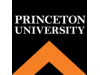list 12个序列
assignment 等级：入门
chat_bubble_outline 语言：英语

-
starstarstarstarstar
0条评论

credit_card 免费进入
timer 60小时总数

## 关于内容

Statistics One is a comprehensive yet friendly introduction to statistics.

more_horiz 查看更多
more_horiz 收起
dns

## 课程大纲

Lecture Topics
• Lecture 1: Experimental research
• Lecture 2: Correlational research
• Lecture 3: Variables and distributions
• Lecture 4: Summary statistics
• Lecture 5: Correlation
• Lecture 6: Measurement
• Lecture 7: Introduction to regression
• Lecture 8: Null Hypothesis Significance Tests (NHST)
• Lecture 9: Central limit theorem
• Lecture 10: Confidence intervals
• Lecture 11: Multiple regression
• Lecture 12: Multiple regression continued
• Lecture 13: Moderation
• Lecture 14: Mediation
• Lecture 15: Group comparisons (t-tests)
• Lecture 16: Group comparisons (ANOVA)
• Lecture 17: Factorial ANOVA
• Lecture 18: Repeated measures ANOVA
• Lecture 19: Chi-square
• Lecture 20 Binary logistic regression
• Lecture 21: Assumptions revisited (correlation and regression)
• Lecture 22: Generalized Linear Model
• Lecture 23: Assumptions revisited (t-tests and ANOVA)
• Lecture 24: Non-parametrics (Mann-Whitney U, Kruskal-Wallis)

Lab Topics:
• Lab 2: Histograms and summary statistics
• Lab 3: Scatterplots and correlations
• Lab 4: Regression
• Lab 5: Confidence intervals
• Lab 6: Multiple regression
• Lab 7: Moderation and mediation
• Lab 8: Group comparisons (t-tests, ANOVA, post-hoc tests)
• Lab 9: Factorial ANOVA
• Lab 10: Chi-square
• Lab 11: Non-linear regression (Binary logistic and Poisson)
• Lab 12: Non-parametrics (Mann-Whitney U and Kruskal-Wallis)

record_voice_over

## 教师

• Andrew Conway - Psychology
store

## 内容设计师Princeton University is a private research university located in Princeton, New Jersey, United States. It is one of the eight universities of the Ivy League, and one of the nine Colonial Colleges founded before the American Revolution.
assistant

## 平台Coursera是一家数字公司，提供由位于加利福尼亚州山景城的计算机教师Andrew Ng和达芙妮科勒斯坦福大学创建的大型开放式在线课程。

Coursera与顶尖大学和组织合作，在线提供一些课程，并提供许多科目的课程，包括：物理，工程，人文，医学，生物学，社会科学，数学，商业，计算机科学，数字营销，数据科学 和其他科目。

5/5

5/5

5/5